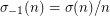# Odd perfect numbers

 Importance: High ✭✭✭
 Author(s): Ancient/folklore
 Subject: Number Theory
 Keywords: perfect number
 Posted by: azi on: September 27th, 2008
Conjecture   There is no odd perfect number.

There is substantial literature on the problem. Most proceeds from a study of the multiplicative functionwhere the conjecture can be stated:implies thatis even.

## Bibliography

* indicates original appearance(s) of problem.

### limiting divisors

My idea is to assume that the OPN is divisible by a prime number (e.x. 3) then use the properties of perfect numbers to figure out other numbers the OPN is divisible by.